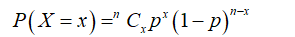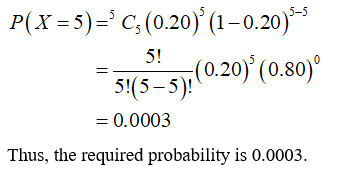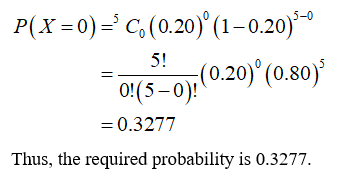# I have a robot and you have a robot and they are going to race each other. The probability that my robot is going to win 0.2 (i.e a 20% chance). We know this because that is how we programmed them. We are going to race them five times.1. What is the probability that my robot wins all the races?2. What is the probability that my robot wins none of the races?3. What is the probability that my robot wins some but not ll of the races?4. What is the probability that my robot wins most of the races?There's more:5. If in addition to the above, there is also a probability (indepndent) that each of our robot's battery will fail before the finish is 0.1 (10%)

Question
1 views

I have a robot and you have a robot and they are going to race each other. The probability that my robot is going to win 0.2 (i.e a 20% chance). We know this because that is how we programmed them. We are going to race them five times.
1. What is the probability that my robot wins all the races?
2. What is the probability that my robot wins none of the races?
3. What is the probability that my robot wins some but not ll of the races?
4. What is the probability that my robot wins most of the races?

There's more:

5. If in addition to the above, there is also a probability (indepndent) that each of our robot's battery will fail before the finish is 0.1 (10%)

check_circle

Step 1

Hello. Since your question has multiple sub-parts, we will solve first three sub-parts for you. If you want remaining sub-parts to be solved, then please resubmit the whole question and specify those sub-parts you want us to solve.

From the provided information,

There is 20% chance that robot is going to win.

The probability of success (p) = 0.20

Sample size (n) = 5

Let x be a random variable which represents the number of time robot wins.

The process will follow binomial distribution as follow:Step 2

1)

The required probability that robot wins all the races can be obtained as:Step 3

2)

The required probability that robot wins none of the races can be obtained as:...

### Want to see the full answer?

See Solution

#### Want to see this answer and more?

Solutions are written by subject experts who are available 24/7. Questions are typically answered within 1 hour.*

See Solution
*Response times may vary by subject and question.
Tagged in